# CLASS 11 MATHS CHAPTER-4 PRINCIPLE OF MATHEMATICAL INDUCTION

Prove the following by using the principle of mathematical induction for all n ∈ N:

Question1.1+3+32+…..+3n-1=(3n-1)/2

Solution :
Letp(n):1+3+32+…..+3n-1 = (3n-1)/2

for n = 1

L.H.S = 31-1 = 1

Hence by Principle of Mathematical Induction,N is true for all n ∈ N.

Question2.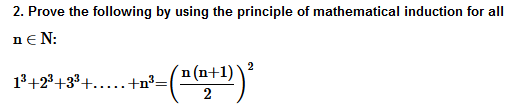Solution :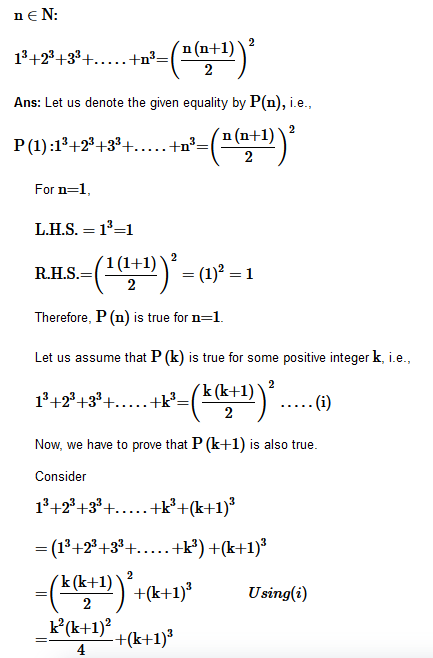Question3.Prove the following by using the principle of mathematical induction for all n∈N .

Solution :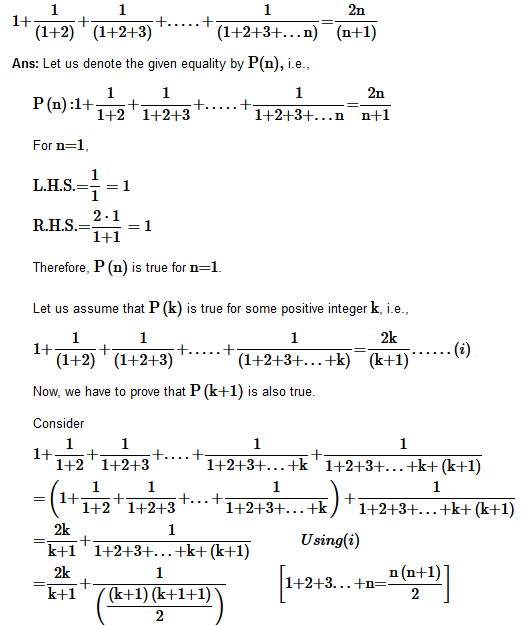Question4. Prove the following by using the principle of mathematical induction for all n∈ N:

1.2.3 +2.3.4 +…+ n(n + 1)(n + 2) = n(n+1) (n+2) (n+3)/4

Solution :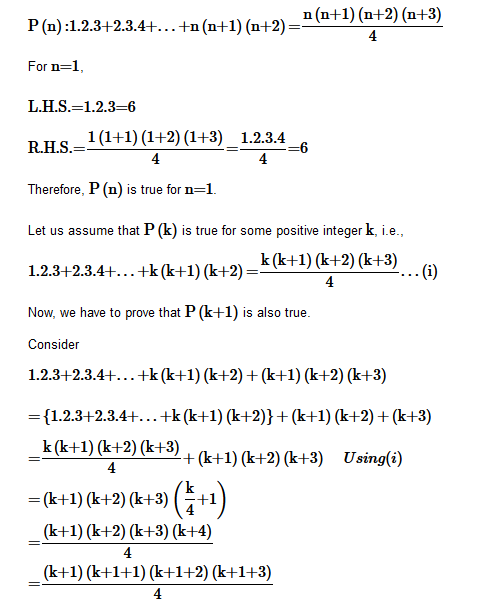Therefore, P(k+1) holds whenever P(k)

holds.

Hence, the given equality is true for all natural numbers i.e., N
by the principle of mathematical induction.

Question5. Prove the following by using the principle of mathematical induction for all n∈ N:

Solution :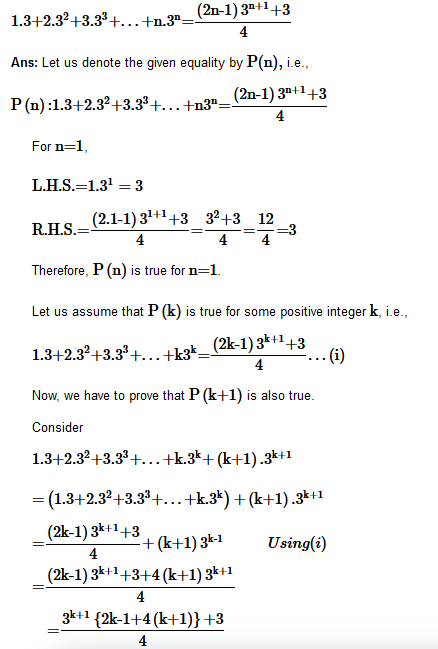Question6. Prove the following by using the principle of mathematical induction for all n∈ N:

Hence, the given equality is true for all natural numbers i.e., N by the principle of mathematical induction.

.

Question7. Prove the following by using the principle of mathematical induction for all n∈ N:

Solution :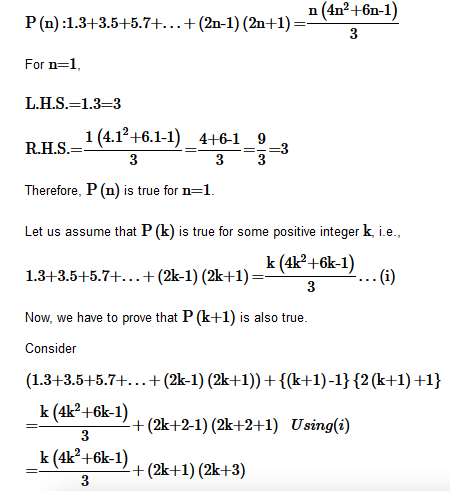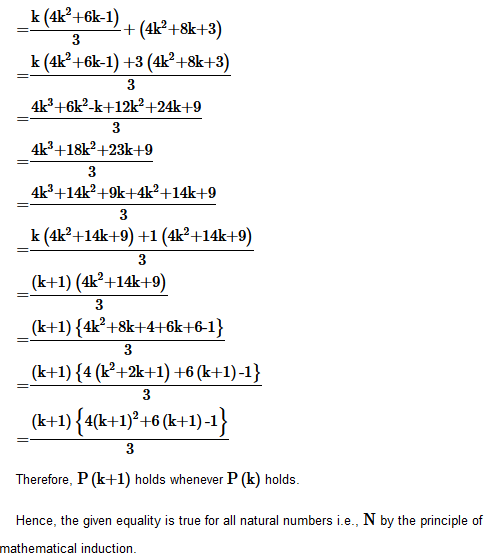Question 8.  Prove the following by using the principle of mathematical induction for all n∈ N:

1.2+2.22+3.22+….+n.2n=(n-1)2n+1+2

Solution :
Let p(n) : 1.2+2.22+3.22+….+n.2n=(n-1)2n+1+2

For n = 1

L.H.S =1.2 = 2

R.H.S.=(1-1)21+1+2=0+2=2

Now, let p(n) be true for n = 1

Let us assume that P(k) is true for some positive integer k, i.e.,

1.2+2.22+3.22+…+k.2k=(k-1)2k+1+2…(i)

Now, we have to prove that P(k+1) is also true.

Consider

{1.2+2.22+3.22+…+k.2k}+(k+1).2k+1

=(k-1)2k+1+2+(k+1)2k+1

=2k+1{(k-1)+(k+1)}+2

=2k+1.2k+2

=k.2(k+1)+1 +2

={(k+1)-1}2(k+1)+1+2

=Therefore, P(k+1) holds whenever P(k)

holds.

Hence, the given equality is true for all natural numbers i.e., N
by the principle of mathematical induction.

Hence by Principle of Mathematical Induction,is true for all n ∈ N.

Question 9. Prove the following by using the principle of mathematical induction for all n∈ N:

Question 10. Prove the following by using the principle of mathematical induction for all n∈ N:

Solution :
Let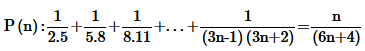For n = 1

Question11. Prove the following by using the principle of mathematical induction for all n∈ N:

Solution :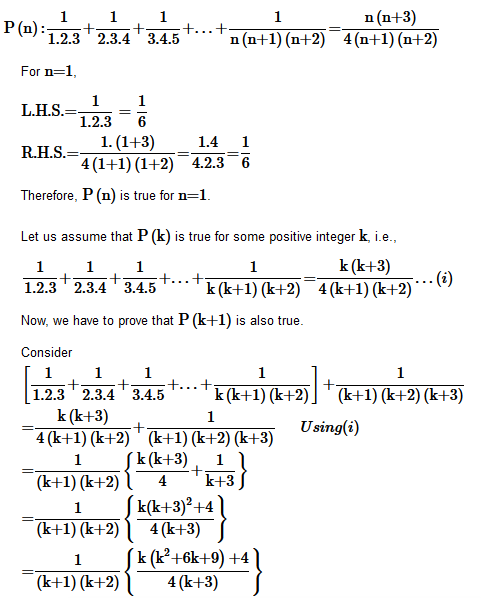Question12. Prove the following by using the principle of mathematical induction for all n∈ N:

Solution :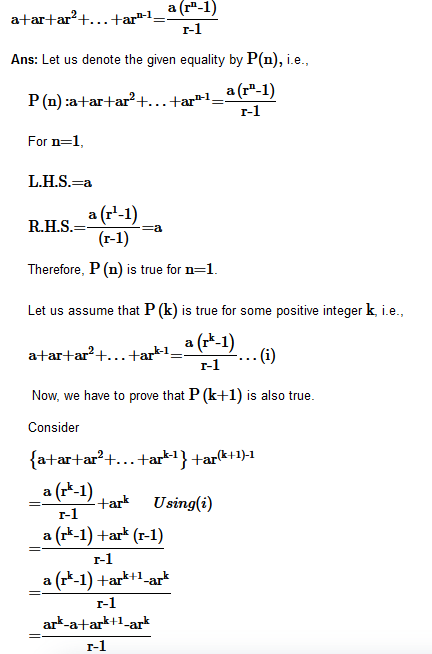Question13. Prove the following by using the principle of mathematical induction for all n∈ N:

Solution :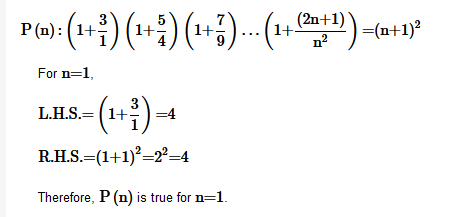Question14. Prove the following by using the principle of mathematical induction for all n∈ N:

Solution :
Let

Question 15. Prove the following by using the principle of mathematical induction for all n∈ N:

Solution :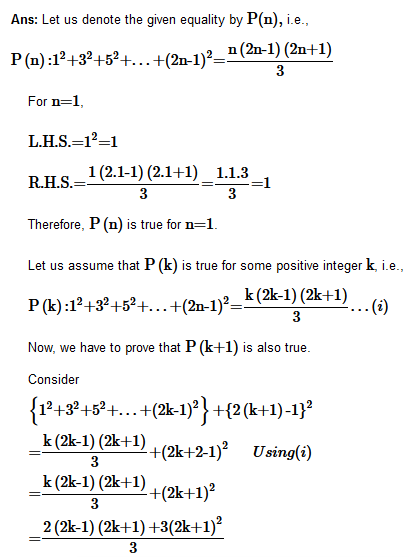Question16.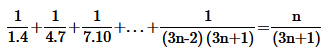Solution :

for n = 1

Therefore, P(k+1) holds whenever P(k)holds.

Hence, the given equality is true for all natural numbers i.e., N
by the principle of mathematical induction.

Question 17. Prove the following by using the principle of mathematical induction for alln∈N: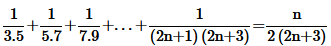Solution :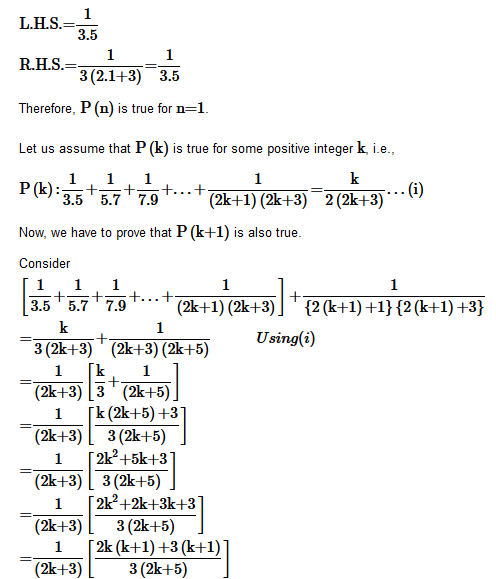Therefore, P(k+1) holds whenever P(k)holds.

Hence, the given equality is true for all natural numbers i.e., N
by the principle of mathematical induction.

Question18. Prove the following by using the principle of mathematical induction for alln∈N: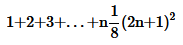Solution :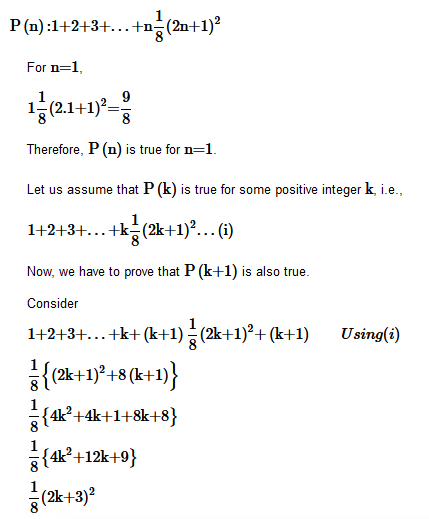Question19. Prove the following by using the principle of mathematical induction for alln∈N:n (n + 1) (n + 5) is a multiple of 3.

Solution :Let us denote the given statement by P(n)
i.e.

P(n):n(n+1)(n+5)  which is a multiple of 3
For n=1
1(1+1)(1+5)=12,
which is a multiple of 3.
Therefore, P(n)
is true for n=1.
Let us assume that P(k)
is true for some natural number k,

k(k+1)(k+5)
is a multiple of 3.
∴k(k+1)(k+5)=3m
, where m∈N
…(i)

Now, we have to prove that P(k+1)
is also true whenever P(k)

is true.

Consider

(k+1){(k+1)+1}{(k+1)+5}

=(k+1)(k+2){(k+1)+5}

=(k+1)(k+2)(k+5)+(k+1)(k+2)

={k(k+1)(k+5)+2(k+1)(k+5)}+(k+1)(k+2)

=3m+(k+1){2(k+5)+(k+2)}

=3m+(k+1){2k+10+k+2}

=3m+(k+1){3k+12}

=3m+3(k+1){k+4}

=3{m+(k+1)(k+4)}=3 × q
, where q={m+(k+1)(k+4)}

is some natural number.

Hence, (k+1){(k+1)+1}{(k+1)+5}
is a multiple of 3

.

Therefore, P(k+1)
holds whenever P(k)

holds.

Hence, the given equality is true for all natural numbers i.e., N
by the principle of mathematical induction.

Question20. Prove the following by using the principle of mathematical induction for alln∈N: 102n-1 + 1 is divisible by 11.

Ans.

Let P(n) : 102n-1 + 1 is divisible by 11.

For n = 1 is divisible by 11

P(1)=102n-1 + 1=11 and P(1) is divisible by 11
Therefore, P(n)
is true for n=1
Let us assume that P(k)
is true for some natural number k
i.e.,

i.e., 102n-1 +1
is divisible by 11

.

∴102k-1 +1 = 11m
, where m∈N

…(i)

Now, we have to prove that P(k+1)
is also true whenever P(k)

is true.

Consider

102(k+1) -1 + 1

=102k+2-1 +1

=102k+1 +1

=102(102k-1+1-1)+1

=102(102k-1+1 -1)-102+1

=102.11m-100+1  Using(i)

=100 × 11m-99

=11(100m-9)

=11r
, where r=(100m-9)

is some natural number

Therefore, 102(k+1)-1+1
is divisible by 11

Therefore, P(k+1)
holds whenever P(k)

holds.

Hence, the given equality is true for all natural numbers i.e., N
by the principle of mathematical induction.

Question 21. Prove the following by using the principle of mathematical induction for alln∈N: x2n –y2n is divisible by x+y.
Solution :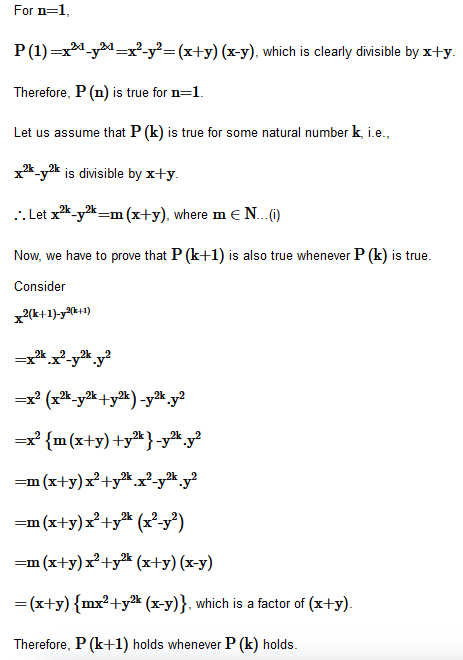Hence, the given equality is true for all natural numbers i.e., N by the principle of mathematical induction.true.

Question22. Prove the following by using the principle of mathematical induction for all n∈N: 32n+2-8n-9
is divisible by 8.

Solution :
Let 32n+2-8n-9 is divisible by 8.

For p(n): 3is divisible by 8 = 64 is divisible by 8

=8r, where r=(9m+8k+8) is a natural number
Therefore,
32(k+1)+2-8(k+1)-9
is divisible by 8
Therefore, P(k+1) holds whenever P(k)
holds.
Hence, the given equality is true for all natural numbers i.e., N
by the principle of mathematical induction.

Question 23. Prove the following by using the principle of mathematical induction for all n∈N: 41– 14is a multiple of 27.

Solution :
Let 41– 14is a multiple of 27.

for  n = 1,

Hence, the given equality is true for all natural numbers i.e., N by the principle of mathematical induction.

Question 24.(2n+7) < (n + 3)2

Solution :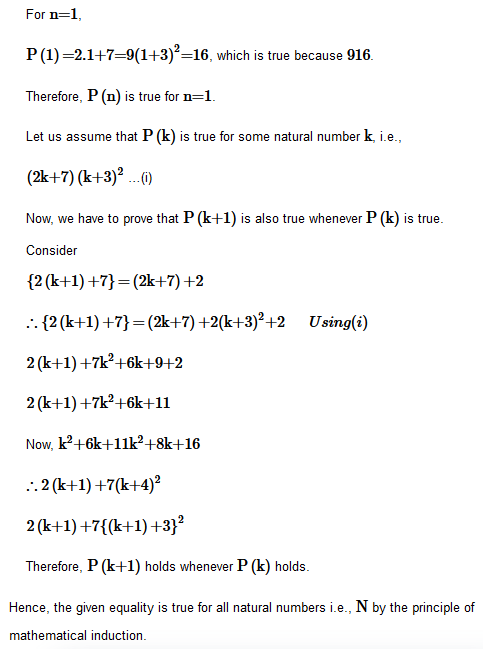Get 30% off your first purchase!

X
error: Content is protected !!
Scroll to Top